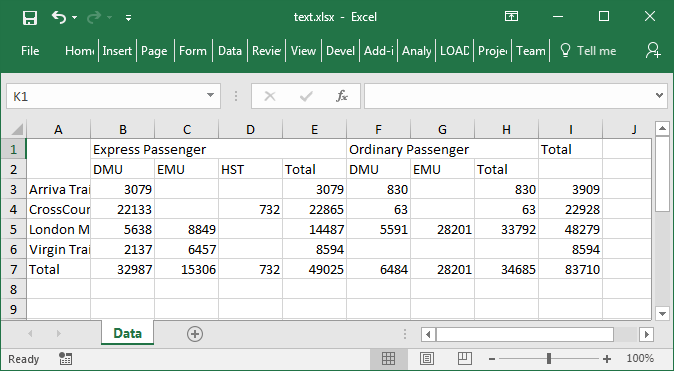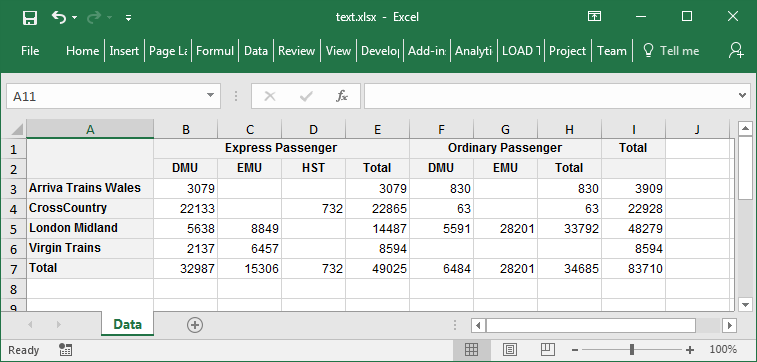# Excel Export

## In This Vignette

• Overview
• Basic Export (no styling)
• Styling and Formatting
• Formatting Cell Values
• Formatting Headings
• Export of NA, NaN, -Inf and Inf
• Column Widths and Row Heights
• Performance
• Styling Reference
• Further Reading

## Overview

The pivottabler package can export a pivot table to an Excel file. Only Excel 2007 onwards (*.xlsx) files are supported. This export uses the openxlsx package.

This vignette starts with a basic (unformatted) export. Following this are various styled/formatted examples.

## Basic Export (no styling)

Exporting a pivot table to an Excel table is reasonably straightforward:

1. Create a pivot table in R using pivottabler,
2. Using the openxlsx package, create a new Excel file and add a worksheet (or open an existing worksheet),
3. Call the writeToExcelWorksheet method on the pivot table.
4. Using the openxlsx package, save the workbook.
library(pivottabler)
pt <- PivotTable$new() pt$addData(bhmtrains)
pt$addColumnDataGroups("TrainCategory") pt$addColumnDataGroups("PowerType")
pt$addRowDataGroups("TOC") pt$defineCalculation(calculationName="TotalTrains", summariseExpression="n()")
pt$evaluatePivot() library(openxlsx) wb <- createWorkbook(creator = Sys.getenv("USERNAME")) addWorksheet(wb, "Data") pt$writeToExcelWorksheet(wb=wb, wsName="Data",
topRowNumber=1, leftMostColumnNumber=1, applyStyles=FALSE)
saveWorkbook(wb, file="C:\\test.xlsx", overwrite = TRUE)Basic Excel Export (unstyled)

## Styling and Formatting

The Excel output from the pivottabler package has been written so that, as much as possible, the same styling used for HTML output also works for the Excel output, i.e. most of the styling described in the Styling vignette can also be used when writing a pivot table to an Excel file.

More specifically, the styling described in the Styling vignette uses CSS (Cascading Style Sheet) definitions for styles. The pivottabler package interprets these CSS definitions and maps them to the styling used in Excel/by the openxlsx package.

This means, once a pivot table has been styled as described in the Styling vignette, the pivot table in the Excel workbook can be styled simply by specifying applyStyles=TRUE.

library(pivottabler)
pt <- PivotTable$new() pt$addData(bhmtrains)
pt$addColumnDataGroups("TrainCategory") pt$addColumnDataGroups("PowerType")
pt$addRowDataGroups("TOC") pt$defineCalculation(calculationName="TotalTrains", summariseExpression="n()")
pt$evaluatePivot() library(openxlsx) wb <- createWorkbook(creator = Sys.getenv("USERNAME")) addWorksheet(wb, "Data") pt$writeToExcelWorksheet(wb=wb, wsName="Data",
topRowNumber=1, leftMostColumnNumber=1,
applyStyles=TRUE, mapStylesFromCSS=TRUE)
saveWorkbook(wb, file="C:\\test.xlsx", overwrite = TRUE)Using CSS Styling

In general, the CSS mappings described above will simplify outputting to Excel. However, not all CSS definitions can be mapped to Excel. Excel also has some style settings that don’t map to CSS. There may also be occasions where different styling is desired in Excel vs. HTML. To support all of these scenarios, a second set of styling properties are also supported. These all begin with “xl-” and have roughly similar (but not exactly the same) names to their CSS counterparts, e.g. the property corresponding to CSS “font-family” is “xl-font-name”. If both the “xl-…” Excel property and the CSS property are specified, the Excel value is used. If mapStylesFromCSS=FALSE is specified, then the CSS properties are ignored and only the “xl-…” properties are used.

The table at the bottom of this vignette details the full set of CSS and Excel style properties that are supported.

## Formatting Cell Values

There are a few different ways for format the values written into the worksheet. These are controlled by the outputValuesAs parameter, which has allowed values: “rawValue”, “formattedValueAsText” and “formattedValueAsNumber”.

The following pivot table will be used to illustrate the different values for this parameter:

library(pivottabler)
library(dplyr)
library(lubridate)

# derive some additional data
trains <- mutate(bhmtrains,
ArrivalDelta=difftime(ActualArrival, GbttArrival, units="mins"),
ArrivalDelay=ifelse(ArrivalDelta<0, 0, ArrivalDelta))

# create the pivot table
pt <- PivotTable$new() pt$addData(trains)
pt$addColumnDataGroups("TrainCategory") pt$addColumnDataGroups("PowerType")
pt$addRowDataGroups("TOC", totalCaption="All TOCs") pt$defineCalculation(calculationName="MeanArrivalDelay", caption="Mean Arr. Delay",
summariseExpression="mean(ArrivalDelay, na.rm=TRUE)", format="%.1f")
pt$renderPivot() ### Raw Value Specifying outputValuesAs="rawValue" will output the raw unformatted values. This is also the default if no value is explicitly specified for the outputValuesAs parameter. library(pivottabler) library(dplyr) library(lubridate) # derive some additional data trains <- mutate(bhmtrains, ArrivalDelta=difftime(ActualArrival, GbttArrival, units="mins"), ArrivalDelay=ifelse(ArrivalDelta<0, 0, ArrivalDelta)) # create the pivot table pt <- PivotTable$new()
pt$addData(trains) pt$addColumnDataGroups("TrainCategory")
pt$addColumnDataGroups("PowerType") pt$addRowDataGroups("TOC", totalCaption="All TOCs")
pt$defineCalculation(calculationName="MeanArrivalDelay", caption="Mean Arr. Delay", summariseExpression="mean(ArrivalDelay, na.rm=TRUE)", format="%.1f") pt$evaluatePivot()

library(openxlsx)
wb <- createWorkbook(creator = Sys.getenv("USERNAME"))
addWorksheet(wb, "Data")

## Export of NA, NaN, -Inf and Inf

The pt\$writeToExcelWorksheet(...) function supports an exportOptions parameter that provides control over how NA, NaN, -Inf and Inf values in R are exported to Excel. See the Appendix: Details vignette for details.

## Column Widths and Row Heights

It is possible to specifying a minimum row height and/or column width as part of the styling. The relevant styling properties are “xl-min-row-height” and “xl-min-column-width”.

Rows/columns are sized to meet all of the minimum sizes specified. E.g. if three cells in the same row have minimum row heights of 40, 45 and 50 specified, the row height will be set to 50.

## Performance

Creating Excel files is relatively effort intensive. Outputting pivot tables to Excel files requires more time than creating a HTML representation of a pivot table. In order of increasing time required:

• Unstyled Pivot Tables - these will be written to Excel quicker than styled pivot tables.
• Pivot tables using only base styles - i.e. where no additional styles have been applied to individual cells in the pivot table - these take a moderate amount of time to write to Excel.
• Pivot tables with styles applied to individual cells - these take the longest amount of time to write to Excel due to the extra effort of handling the styles applied to individual cells.

## Styling Reference

A reference table of the supported styling declarations can be found in the Appendix: Details vignette.

## Further Reading

The full set of vignettes is: